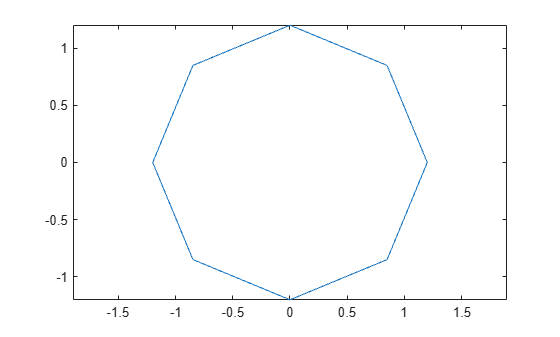# polyarea

Area of polygon

## Syntax

``a = polyarea(x,y)``
``a = polyarea(x,y,dim)``

## Description

example

````a = polyarea(x,y)` returns the area of the 2-D polygon defined by the vertices in vectors `x` and `y`. If `x` and `y` are vectors of the same length, then `polyarea` returns the scalar area of the polygon defined by `x` and `y`.If `x` and `y` are matrices of the same size, then `polyarea` returns a row vector containing the areas of each polygon defined by the columnwise pairs in `x` and `y`. If `x` and `y` are multidimensional arrays, then `polyarea` operates along the first dimension whose length is not equal to 1. ```
````a = polyarea(x,y,dim)` operates along dimension `dim`.```

## Examples

collapse all

Create a set of points that define a 2-D polygon.

```p = linspace(0,2.*pi,9); x = 1.2*cos(p)'; y = 1.2*sin(p)'; ```

Plot the polygon.

```plot(x,y); axis equal```Compute the area of the polygon.

`a = polyarea(x,y)`
```a = 4.0729 ```

## Input Arguments

collapse all

x-coordinates, specified as a vector, matrix, or multidimensional array.

y-coordinates, specified as a vector, matrix, or multidimensional array.

Dimension to operate along, specified as a positive integer scalar.

## Tips

• The area value returned by `polyarea` can depend on the order of the input points.

## Version History

Introduced before R2006a